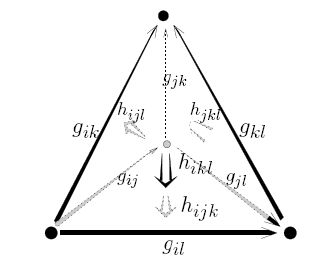## Classifying Spaces for Topological 2-GroupsCategorifying the concept of topological group, one obtains the notion of a topological 2-group. This in turn allows a theory of "principal 2-bundles" generalizing the usual theory of principal bundles. It is well-known that under mild conditions on a topological group G and a space M, principal G-bundles over M are classified by either the Cech cohomology H1(M,G) or the set of homotopy classes [M,BG], where BG is the classifying space of G. Here we review work by Bartels, Jurco, Baas-Bökstedt-Kro, Stevenson and others generalizing this result to topological 2-groups. We explain various viewpoints on topological 2-groups and the Cech cohomology H1(M,G) with coefficients in a topological 2-group G, also known as "nonabelian cohomology". Then we sketch a proof that under mild conditions on M and G there is a bijection between H1(M,G) and [M,B|G|], where B|G| is the classifying space of the geometric realization of the nerve of G.

Click on this to see the transparencies of the talk:

• Classifying Spaces for Topological 2-Groups - in PDF and Postscript
For a less technical version with more applications, try this:
• Classifying Spaces for Topological 2-Groups - in PDF and Postscript
These talks summarize the following paper:
which in turn is based on the following work:
See also these related talks, which cover other aspects of the big picture: